﻿ A Method for Teaching How to Balance Redox Reactions by Building Up MoleculesPublications are Open
Access in this journal
Article Versions
Export Article
• Normal Style
• MLA Style
• APA Style
• Chicago Style
Research Article
Open Access Peer-reviewed

### A Method for Teaching How to Balance Redox Reactions by Building Up Molecules

Balakrishnan Viswanathan , Mohamed Shajahan Gulam Razul
World Journal of Chemical Education. 2020, 8(2), 67-70. DOI: 10.12691/wjce-8-2-2
Received February 03, 2020; Revised March 11, 2020; Accepted March 24, 2020

### Abstract

The standard first-year program includes a section on the balancing of chemical reactions. Students find it difficult to understand this concept as it is increasingly presented as an algorithm or mathematical procedure. This submission outlines a method of teaching students how to balance redox reactions using known chemical principles: oxidation state (including the ionic approximation of molecules), conservation of number (conservation of mass and non-transmutability of chemical particles), and spectator ions. The method involves identification of the oxidation/reduction pairs and balancing the skeletal oxidation/reduction reactions (electron loss/gain) to enforce the link between change in oxidation state and transfer of electrons. The molecular species in the reaction are then built up by adding in other elements with their associated oxidation states, treating them as spectator ions. Equalising the number of electrons in the oxidation and reduction reactions leads to the balanced redox reaction. This method has been tested on a variety of reactions and examples of the three classes of redox reactions are presented. It is expected that students will be able to master the title concept due to the link to fundamental chemical concepts, further enhancing their understanding of the concepts involved.

### 1. Introduction

The balancing of redox reactions is part of every first-year chemistry program. The goal behind this exercise is to extend the basic concepts of stoichiometry to consider electrons (in addition to atoms). In this manner, students are encouraged to think of electrons as objects that can be transferred between species. This has the potential to show students that electrons are an integral part of reactivity. This then provides a natural (conceptual) link to electron structure and Lewis structures.

Over the years, this important concept has evolved from being a chemical concept to be understood to an algorithmic procedure to be mastered. 1 As a result of this evolution, the methodologies developed for balancing reactions have been more mathematical 2, 3 and hence, more chemically abstract. This increase in abstraction has led to students finding it difficult to master the concept. 4 This is not surprising since the trend toward mathematics reduces the connection to chemical principles, which is seen in the disconnect 5 between students’ ability to solve algorithmic problems and their ability to explain the underlying concepts. The increase in abstraction is also undesirable because it disproportionately disadvantages students who lack a strong mathematical background. 6 Hence, facility in mathematics effectively acts as a gatekeeper for success in stoichiometry. The description of redox equations as systems to be solved algorithmically hides the involved chemical concepts from students and the exercise is reduced to rote memorisation of an algorithm 7.

The “half-reaction” approach 8 to balancing redox reactions identifies the species that undergo oxidation and reduction (and their corresponding products) by computing the oxidation states of all elements in species. The following steps are applied to each half-reaction,

1. The elements other than oxygen and hydrogen are balanced by inspection.

2. The oxygen atoms are balanced using water molecules.

3. The hydrogen atoms are balanced using H+ ions.

4. The total charge is balanced using electrons.

The above method clearly assumes that the reaction occurs in water; this is a reasonable assumption at the first-year level. More importantly, electron transfer is the last step in the process, whereas electron transfer is the very reason for the redox reaction. This separation (of steps) between oxidation/reduction and electron transfer leads to separation between the two concepts in the minds of the students. The balancing of electrons is no longer connected to the change in oxidation state.

The principles underlying the balancing of reactions (redox or otherwise) are foundational in chemistry: mass conservation, non-transmutability of chemical particles (atoms and electrons), and transfer of electrons between oxidised and reduced species. It is therefore important that students be encouraged to engage with these concepts to address the issue of stoichiometry, since stoichiometry is at the heart of chemistry. Resorting to “inspection” 1 and abstract mathematical algorithms do little to improve students’ understanding of fundamental chemical principles; the focus needs to be on chemistry. Teaching requires implicit concepts to be made explicit. Mastery is attained when the student can make explicit concepts implicit.

### 2. Proposed Method

Redox reactions are reactions in which two or more atoms experience changes in their oxidation states. IUPAC defines the oxidation state of an atom as the “charge of this atom after ionic approximation of its heteronuclear bonds”. 9 Under this definition, all atoms in a polyelemental molecule are described as ions. The change in oxidation state is thus a change in charge on the ion in a molecule. Students are typically aware of the concept of “spectator ions” by the time redox chemistry (especially redox stoichiometry) is introduced.

In the proposed method the concept of ‘spectator ions’ is extended to sub-molecular ions (atoms assigned charges equal to their oxidation states). Molecules are therefore made of ‘ions’, in keeping with the IUPAC definition of oxidation state. It is then possible to explicitly represent a half-reaction as the gain or loss of electrons by atoms of an element. This explicitly reinforces the relationship between oxidation/reduction and electron transfer. The oxidised/reduced half-reaction is completed by adding in sufficient ‘spectator ions’ (equal numbers on both sides of the equation to ensure balance) to complete all species on both sides of the equation. The procedure can be summarised by the following steps (applied equally to oxidation and reduction half-reactions):

1. The oxidised/reduced element (left side of the equation) and the corresponding reduced/oxidised species (right side) are identified from the oxidation states. This forms the skeleton half-reaction.

2. Electrons are added to balance charge.

3. Sufficient ‘spectator atoms’ (with their oxidation numbers as charges) are added equally to both sides of the skeleton oxidation half-reaction in order to build up molecules/ions. This yields the half-reaction.

4. Sufficient H+ is added (equally to both sides of the reaction) to convert all

a. O2- to water (acidic conditions)

b. O2- to [OH]- (basic conditions)

c. Unbalanced H+ is neutralised to water by adding [OH]- (basic conditions).

As in the traditional method, the oxidation and reduction half-reactions are multiplied by suitable factors to equalise the number of electrons transferred. Subsequently, the two reactions are added and the resultant is simplified by cancelling species present on both sides of the equation.

This method derives from the concept that the elements oxidised/reduced within a molecule do not affect the other atoms present. These other atoms can be considered to be spectator atoms since their oxidation numbers do not change during the reaction. For this reason, we can add them separately on both sides of the equation. Then we can build up the molecules and polyatomic ions that participate in the reaction. By adding the same species in equal quantities to both sides of the reaction, we ensure that every step is balanced, thus reinforcing the conservation of numbers principle and minimising working errors.

### 3. Examples

Mousavi 10 has described three classes of redox reactions and presented approaches to balancing them. Class I reactions are those in which one molecular species undergoes both oxidation and reduction; this situation is seen in electrolysis reactions. Class II reactions are defined as reactions in which one element has multiple oxidation states on the right-hand side of the equation; these are typical of disproportionation reactions. Class III encompasses all redox reactions that are not covered under Classes I and II. These three situations will be treated using the current method below. For the sake of clarity, the reactions considered by Mousavi 10 are explored here.

3.1. Class I Reactions

This class of reactions involves one reactant and multiple products. An example is the electrolysis of water.(1)

The oxidation states of the elements involved in this reaction are given below.

The skeletal oxidation half-reaction is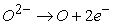(2)

This reaction is doubled to account for the number of oxygen atoms in the right-hand side (the oxygen molecule) of Equation 1.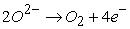(3)

Next, the molecules on the left-hand side of the reaction are built up by adding H+.(4)

Equation 4 is the oxidation half-reaction.

The skeletal reduction half-reaction is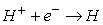(5)

This reaction is doubled to make up the hydrogen molecule.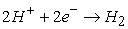(6)

Equation 6 is the reduction half-reaction. Equation 6 must be doubled to equalise the numbers of electrons in the oxidation and reduction half-reactions.(7)(8)

Cancelling common terms leads to the net reaction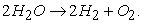(9)
3.2. Class II Reactions

In this class of reactions at least one element is present in two oxidation states on the right-hand side of the reaction. An example of this class of reaction is the oxidation of sodium metal in water, forming sodium hydroxide and liberating hydrogen.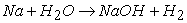(10)

The oxidation states of the elements are

In this reaction, sodium is being oxidised and hydrogen is being reduced. The skeletal oxidation half-reaction is(11)

The species on the right-hand side is completed by adding one oxygen atom (2- oxidation state) and one hydrogen atom (1+ oxidation state). This yields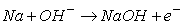(12)

This is the oxidation half-reaction.

The skeletal reduction half-reaction is(13)

This reaction must be doubled to yield hydrogen gas.(14)

This is the reduction half-reaction.

To combine the oxidation and reduction half-reactions, the number of electrons transferred must be equalised. Hence, Equation 12 must be doubled before addition to Equation 14. The resulting equation is (keeping all terms)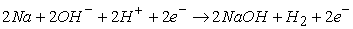(15)

Cancelling common terms and recognising that H+ and [OH]- combine to form water, the final balanced equation is obtained.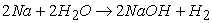(16)
3.3. Equations that Fit both Classes I and II

This is a category that contains a large number of reactions. 10 One example of this type of reaction is the interaction between lead(IV) oxide and an acid to produce a lead(II) salt while evolving oxygen gas.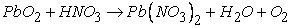(17)

The typical first-year undergraduate would be able to identify that the nitrate ion acts as a spectator in this reaction. The active reaction is(18)

The oxidation states of the elements in this reaction are given in the table below.

In this reaction, oxygen is oxidised and lead is reduced. The skeletal oxidation half-reaction is(19)

This equation must be doubled to form oxygen gas. Adding in Pb4+ to complete the molecule (PbO2 on the left-hand side),(20)

This is the oxidation half-reaction.(21)

Completing the molecule (PbO2 on the left-hand side) leads to(22)

This is the reduction half-reaction.

Doubling Equation 22 (to equalise electrons) and adding it to Equation 20 yields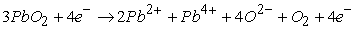(23)

Cancelling common terms (and recognising that Pb4+ + 2O2- → PbO2) leads to(24)

The oxide ions in the above reaction are converted to water using H+ ions to yield the balanced equation.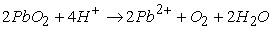(25)

This reaction can also be balanced without identifying that the nitrate ion is a spectator. However, it is natural to apply the knowledge of spectator ions since that very concept is used to build up the molecules. More importantly, Equation 24 shows that the reaction occurs due to the acidic nature of the solution (the acid is required) and hence would occur when any other acid is used. This chemical insight is valuable and can be obtained as a by-product of the process of balancing the equation.

3.4. Class III Reactions

Class III refers to the redox reactions that are not classified under Classes I or II. This is possibly the easiest class of reactions to balance since it represents unique oxidation and reduction pairs. The dissolution of cinnabar in aqua regia is an example.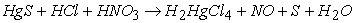(28)

The oxidation states of the elements are presented below.

The skeletal oxidation half-reaction is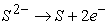(29)(30)

The product is completed by adding sufficient H+ and Cl-, which are spectators to the redox process.(31)

This is the oxidation half-reaction.

The skeletal reduction half-reaction involves the reduction of nitrogen(V) to nitrogen(II).(32)

Sufficient O2- and H+ are added to form HNO3; this also satisfies the necessity for NO.(33)

The oxide ions on the right-hand side are converted to water by adding sufficient H+.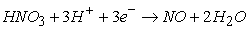(34)

This is the reduction half-reaction.

To equalise electrons, Equation 31 must be multiplied by 3 and Equation 34 by 2. Adding the resultant equations yields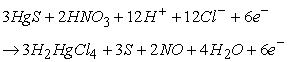(35)

Cancelling common terms and identifying that H+ and Cl- make HCl, the final balanced equation is obtained.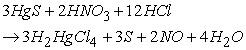(36)

### 4. Conclusions

Oxidation state can be used by first-year undergraduate students to balance even complex redox reactions without needing to memorise complex algorithms; the requirement is that they be able to identify the oxidation and reduction pairs. Learning is enhanced when the complexity of the concept(s) is reduced and connected to concepts that are already known (context). 11 A method that only relies on chemical principles without resorting to complex mathematical procedures is presented. In the method outlined and exemplified here, the change in oxidation number is directly linked to the number of electrons required for the process. This approach takes advantage of the approximation involved in the definition of oxidation state (ionic approximation) and the concept of spectator ions to enable students to internalise the definitions of oxidation and reduction as the loss and gain of electrons, respectively. It is important to explicitly relate oxidation/reduction to the atoms being oxidation/reduction since a large proportion of students incorrectly identifies the species involved in electron transfer. 7 It is expected that educators will find this approach helpful in improving the understanding of chemical concepts by their students.

### References

  W.B. Jensen, Balancing Redox Equations, J. Chem. Educ. 86 (2009) 681. In article View Article  I.B. Risteski, A New Singular Matrix Method for Balancing Chemical Equations and Their Stability, J. Chinese Chem. Soc. 56 (2009) 65-79. In article View Article  M.S. Fox, On Balancing Acidic and Basic Reduction/Oxidation Reactions with a Calculator, World J. Chem. Educ. 3 (2015) 74-77. In article  F. Marais, S. Combrinck, An Approach to Dealing with the Difficulties Undergraduate Chemistry Students Experience with Stoichiometry, South African J. Chem. 62 (2009) 88-96. In article  T.A. Holme, C.J. Luxford, A. Brandriet, Defining Conceptual Understanding in General Chemistry, J. Chem. Educ. 92 (2015) 1477-1483. In article View Article  V.R. Ralph, S.E. Lewis, Chemistry topics posing incommensurate difficulty to students with low math aptitude scores, Chem. Educ. Res. Pract. 19 (2018) 867-884. In article View Article  A.R. Brandriet, S.L. Bretz, Measuring meta-ignorance through the lens of confidence: examining students’ redox misconceptions about oxidation numbers, charge, and electron transfer, Chem. Educ. Res. Pract. 15 (2014) 729-746. In article View Article  T.L. Brown, H.E. LeMay Jr., B.E. Bursten, C.J. Murphy, P.M. Woodward, M.W. Stoltzfus, M.W. Lufaso, Chemistry: The Central Science, 14th ed., Pearson, New York, New York, 2018. In article  M. Nič, J. Jirát, B. Košata, A. Jenkins, A. McNaught, eds., IUPAC Compendium of Chemical Terminology, 2nd ed., IUPAC, Research Triagle Park, NC, 2009. In article View Article  PubMed  A. Mousavi, Revival of the oxidation number method for balancing redox equations, Trans. R. Soc. South Africa. 73 (2018) 86-89. In article View Article  M.M.W. Cheng, Students’ visualisation of chemical reactions – insights into the particle model and the atomic model, Chem. Educ. Res. Pract. 19 (2018) 227-239. In article View Article

Published with license by Science and Education Publishing, Copyright © 2020 Balakrishnan Viswanathan and Mohamed Shajahan Gulam RazulThis work is licensed under a Creative Commons Attribution 4.0 International License. To view a copy of this license, visit http://creativecommons.org/licenses/by/4.0/